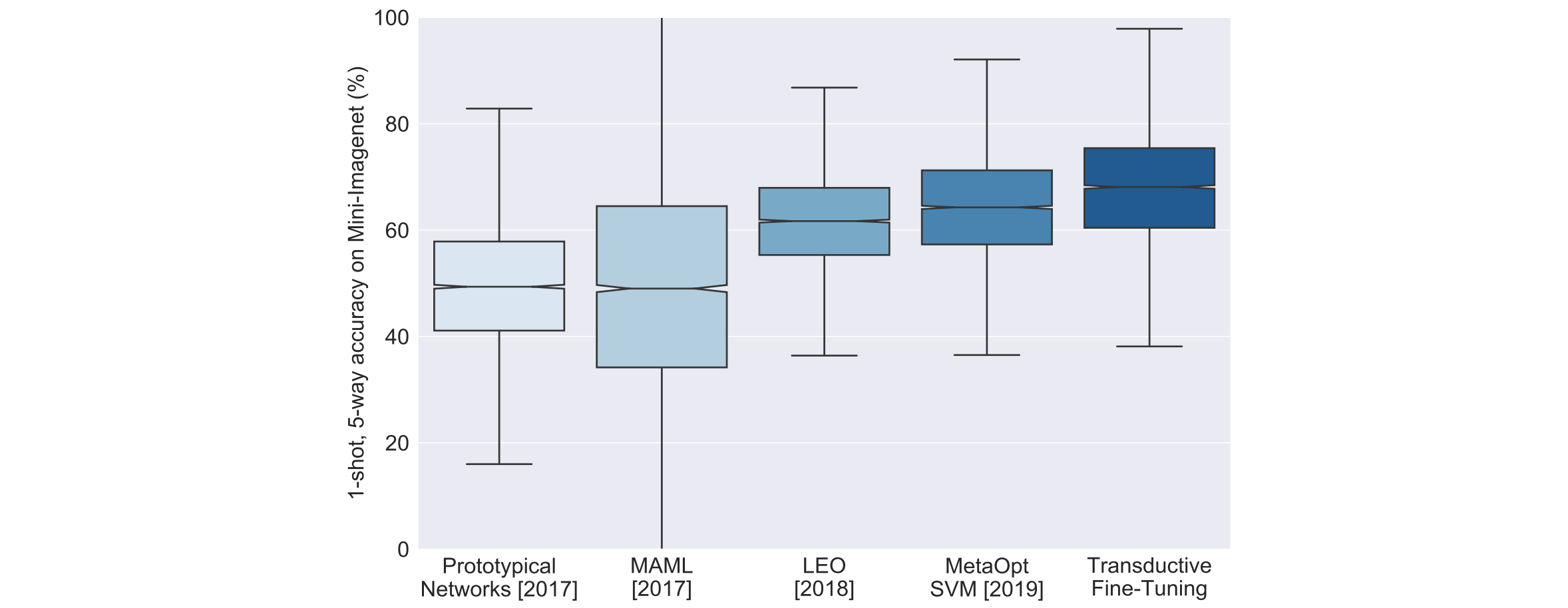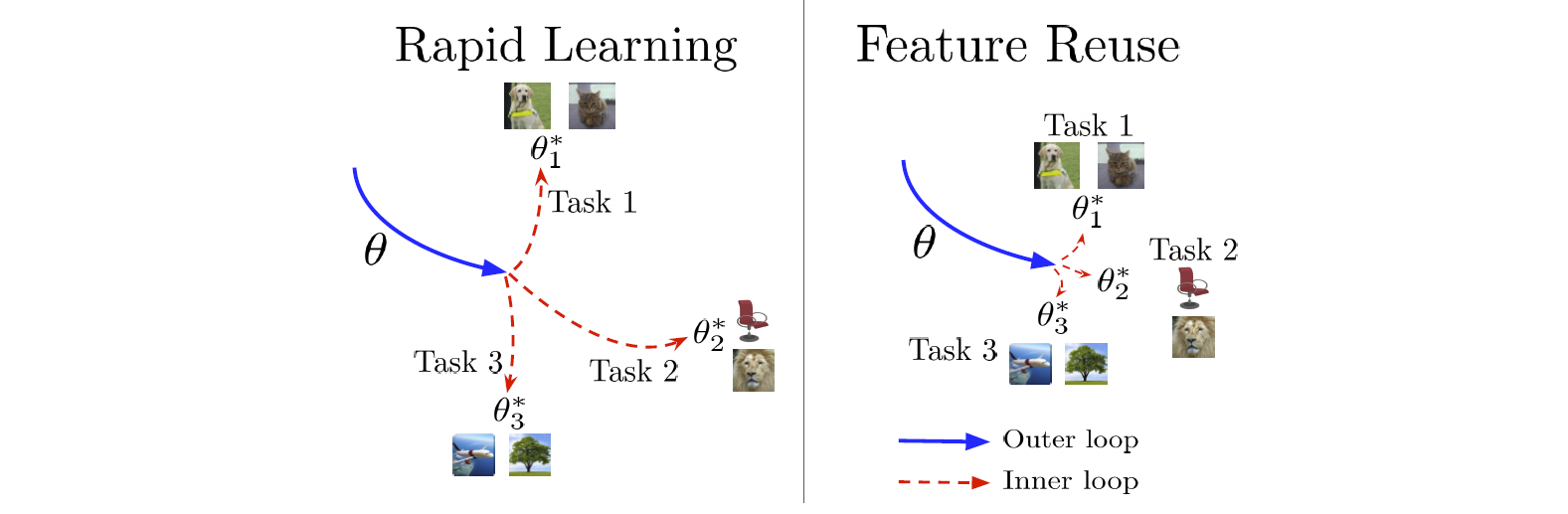Jarvis' Blog (总有美丽的风景让人流连) 总有美丽的风景让人流连

# 关于少样本学习的思考 (二) (Thinking About FSL 2)

2021-03-22
Jarvis
Post

## 1. (ICLR 2020) A Baseline for Few-Shot Image ClassificationTransductive learning (直推学习) 是相对于 inductive learning (归纳学习) 来说的.

• Inductive learning 是指从训练集中归纳出一定的规则(模型), 然后把该规则应用到测试数据上得到结果;
• Transductive learning 指的是直接从训练数据和测试数据中直推出测试的结果, 因此 transductive learning 中是允许测试样本参与模型的构建的.

### 1.1 方法

$\mathbb{R}^{\vert C_t\vert} \ni z(x;\Theta) =w\frac{z(x;\theta)_+}{\Vert z(x;\theta)_+\Vert} + b,$

• Transductive Fine-Tuning

$\Theta^* = \underset{\Theta}{\arg\min}\frac{1}{N_s}\sum_{(x,y)\in\mathcal{D}_s}-\log p_{\Theta}(y\vert x) + \frac{1}{N_q}\sum_{(x,y)\in\mathcal{D}_q}\mathbb{H}(p_{\Theta}(\cdot\vert x)).$

## 2. (ICLR 2020) Rapid Learning or Feature Reuse? Towards Understanding the Effectiveness of MAML• 在 MAML 训练完后, 测试时冻结 meta-learner (即禁用内循环); 然后使用特征相似度评估方法 (Canonical Correlation Analaysis, CCA) 检查内循环在测试时发生了多大的变动.

• 结果显示实际上只有最后的 head layer 参数有显著的变化, 其他层的参数变动非常小. 说明了 MAML 的效果来源于 meta-learner 的特征重用.
• 基于该实验发现, 提出了 ANIL (Almost No Inner Loop) 的方法, 在训练和测试时移除绝大部分的内循环, 仅仅保留最后一层参数的内循环更新.

• 结果显示 ANIL 实现了和 MAML 一样的精度, 而训练和推断速度有了显著的提升.

• 测试时 head layer 的内循环可以去掉而不影响精度, 称为 NIL (No Inner Loop).

• 训练时 head layer 的内循环可以去掉, 否则会显著影响精度.

Content